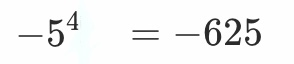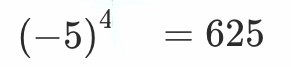# Positive or negative symbolsCan anyone tell me how can brackets change the sign of answer and how to solve them without calculator?

Hi

In the first case we have the operation : - 5 * 5 * 5 * 5 = - 625
In the second case we have a parenthesis so the operation is:
(-5) * (-5) * (-5) * (-5) = 625

If we multiply a negative by a positive it gives a negative value
A positive by a positive gives a positive value
A negative by a negative gives a positive value

1 Like

Got it, Thank you so much🤍